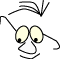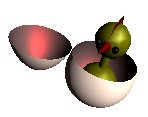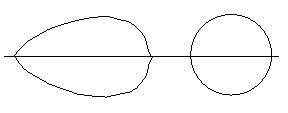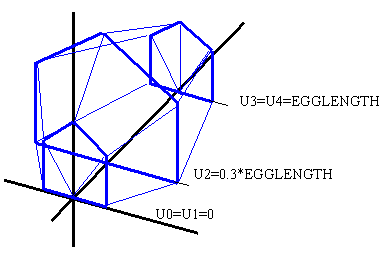DialogboksJOGL>Egg

# Egg

WhatDrawing an egg by combining Bezier surfaces

The task is simply to draw an egg. We will draw the egg closed or opened, and with and without a chicken inside.

We will start by considering the form of an egg.We assume that we can render the egg as round, circular, along one axes. Along the length of the egg however we must look for a form that is different. We should be able to model this form by a polygon. One solution seems to be to describe the egg by a series of circles of varying diameters and connect points on the circles to the neighboring circles. This should give us a mask of as small surfaces we want. We could then use glBegin(GL_TRIANGLE_STRIP) to render the surface of the egg, with GL_SMOOTH turned on. This will be similar to the method used in Torus.

We will however try to model the egg in an other way, by means of a Bezier surface.

## Bezier surfaces

We can base our planning by recognizing the symmetry of an egg. The form is symmetrical with respect to any plane along the length of the egg through it center. This must be useful when we try to formulate a Bezier surface.

We start out by considering the two actual contours of the egg. You can experiment with two simple cubical Bezier curves on the applet below:

As you can see there are some restrictions on the design you can make. The restrictions make sure that the derivats are perpendicular on the axes to assure that we can connect the two halfs of the egg in a continous way.

We know from the mathematics involved that we will have a problem with making a perfect circle by connecting two cubical Bezier curves. We can, however get "close enough" for a practical purpose. means of two

We see that we have an other problem. we can not get the control points along the two axes to produce a usefull circle in one direction and an eggform in the other direction

## A Bezier surface

we will attempt to solve the problem by using more than 4 controlpoints. We will try to describe a "hull" that we can experiment with..The data for this hull:

```// dimensions and controlpoints
float EGGLENGTH    =7.0f;
float ctr1=0.3f*EGGLENGTH;
float ctr2=0.7f*EGGLENGTH;
float ctr3=0.3f*EGGLENGTH;
// offset along z
float ctz1=0.0f*EGGLENGTH;
float ctz2=0.3f*EGGLENGTH;
float ctz3=1.0f*EGGLENGTH;
float ctz4=EGGLENGTH;
// factor for circlecompensation
float rf=1.35f;
//M[UN][VN]
float []M=    // ctrl point map
{
//u=0 -> endpoint z=0
0.0f,    0.0f,    0.0f,//v=0
0.0f,    0.0f,    0.0f,//v=1
0.0f,    0.0f,    0.0f,//v=2
0.0f,    0.0f,    0.0f,//v=3
0.0f,    0.0f,    0.0f //v=4
,
//u=1
ctr1,    0.0f,    ctz1,//v=0
ctr1,    ctr1,    ctz1,//v=1
0.0f,    rf*ctr1,    ctz1,//v=2
-ctr1,    ctr1,    ctz1,//v=3
-ctr1,    0.0f,    ctz1 //v=4
,
//u=2
ctr2,    0.0f,    ctz2,//v=0
ctr2,    ctr2,    ctz2,//v=1
0.0f,    rf*ctr2,    ctz2,//v=2
-ctr2,    ctr2,    ctz2,//v=3
-ctr2,    0.0f,    ctz2 //v=4
,
// u=3
ctr3,    0.0f,    ctz3,//v=0
ctr3,    ctr3,    ctz3,//v=1
0.0f,    rf*ctr3,    ctz3,//v=2
-ctr3,    ctr3,    ctz3,//v=3
-ctr3,    0.0f,    ctz3 //v=4
,
//u=4  -> endpoint z=EGGLEN
0.0f,    0.0f,    ctz4,//v=0
0.0f,    0.0f,    ctz4,//v=1
0.0f,    0.0f,    ctz4,//v=2
0.0f,    0.0f,    ctz4,//v=3
0.0f,    0.0f,    ctz4 //v=4

};
```

The interesting values that we can manipulate are:

```
ctr1=0.3f*EGGLENGTH;
ctr2=0.7f*EGGLENGTH;
ctr3=0.3f*EGGLENGTH;
// offset along z, as U on drawing
ctz1=0.0f*EGGLENGTH;
ctz2=0.3f*EGGLENGTH;
ctz3=1.0f*EGGLENGTH;
ctz4=EGGLENGTH;
// factor for circlecompensation
rf=1.35f;

```

## A complete egg

Give this datadescription a simple egg may be rendered like this:

```void drawSimpleEgg(GL gl)
{
gl.glPolygonMode(GL.GL_FRONT_AND_BACK,GL.GL_FILL);
gl.glLightModeli(GL.GL_LIGHT_MODEL_TWO_SIDE,1);
stdMaterials.setMaterial(gl,stdMaterials.MAT_WARM_WHITE,GL.GL_FRONT);
stdMaterials.setMaterial(gl,stdMaterials.MAT_RED_RUBBER,GL.GL_BACK);
gl.glMap2f(GL.GL_MAP2_VERTEX_3,
0.0f,1.0f,3,UN,
0.0f,1.0f,3*UN,VN,
M,0);
gl.glEnable(GL.GL_MAP2_VERTEX_3);
gl.glEnable(GL.GL_AUTO_NORMAL);
gl.glEnable(GL.GL_NORMALIZE);
gl.glMapGrid2f(40,0.0f,1.0f,40,0.0f, 1.0f);
gl.glFrontFace(GL.GL_CW);
gl.glEvalMesh2(GL.GL_FILL, 0, 40, 0, 40);
// other half
gl.glRotatef(180.0f,0.0f,0.0f,1.0f);
gl.glEvalMesh2(GL.GL_FILL, 0, 40, 0, 40);
gl.glFrontFace(GL.GL_CCW);
}```

## Hat off

We want to take "the hat of the egg". We solve this by drawing the egg twice with a clip plane. That will involve the Bezier surface 4 times. OpenGL lets us define temporary clipping planes when we render a model. The algorithm is based on a z-axes along the length of the egg. A plane is defines as ax+by+cz+d=0. Coefficients a,b,c describes the normal to the plane. d is determined by setting a point in the plane (x,y,z) into the equation. a,b,c,d is set by the function glClipPlane.

We se from the code below that the plane normal coincides with the negative z-axes and has the distance CutOff from the xy-plane.

```void drawEggWithHatOff(GL gl)
{
float CutOff=0.6f*EGGLENGTH;
double[] cutplane=new double[]{0.0f,0.0f,-1.0f,CutOff};
gl.glClipPlane(GL.GL_CLIP_PLANE2,cutplane,0);
gl.glEnable(GL.GL_CLIP_PLANE2);
drawSimpleEgg(gl);
gl.glTranslatef(0.0f,0.0f,EGGLENGTH);
gl.glRotatef(180.0f,1.0f,0.0f,0.0f);
gl.glTranslatef(6.0f,0.0f,0.0f);
cutplane=1.0f;
cutplane=-CutOff;
gl.glClipPlane(GL.GL_CLIP_PLANE2,cutplane,0);
gl.glEnable(GL.GL_CLIP_PLANE2);
drawSimpleEgg(gl);
gl.glDisable(GL.GL_CLIP_PLANE2);
}```

## Chicken

For no special reason we render a chicken when the egg is opened:

_oneEgg.java
References
• The program project: https://svn.hiof.no/svn/psource/JOGL/egg
Maintainance

B.Stenseth, april 2009

(Welcome) JOGL>Egg (Horse)